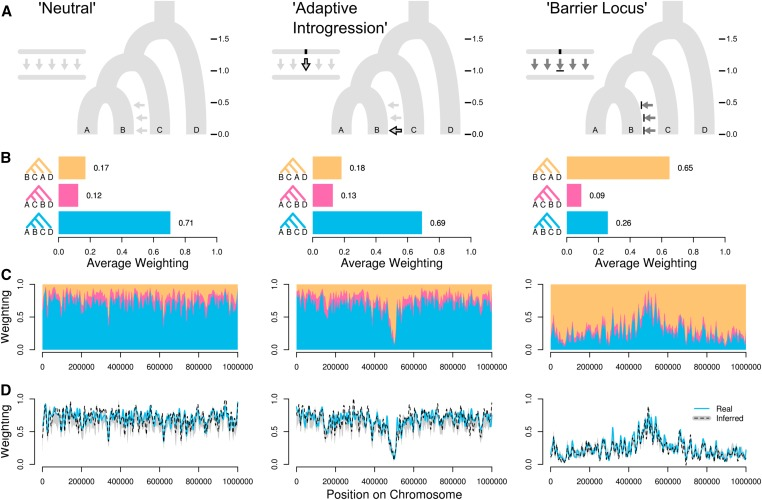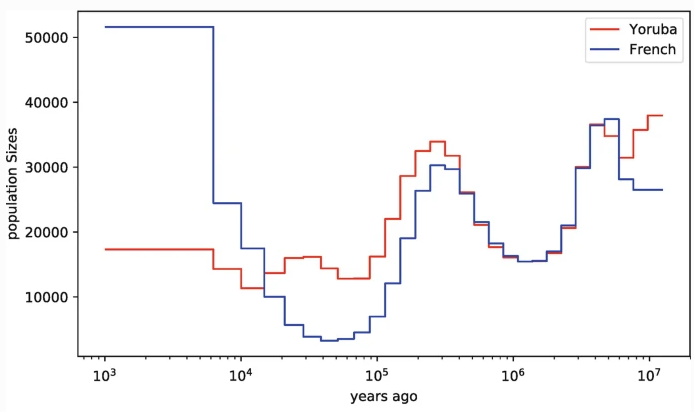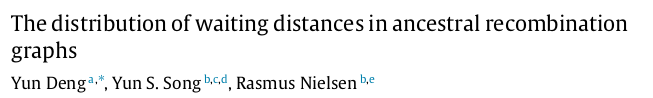## Linking Phylogenetic Inference at Genome-wide and Genealogical Scales### Genealogical variation

Genomes are composed of a mosaic of segments inherited from different ancestors,
each separated by past recombination events.Consequently, genealogical relationships vary spatially across genomes.

### Multispecies coalescent assumptions

The multispecies coalescent (MSC) describes the expected distribution of unlinked genealogies, as a function of demographic model parameters (N$_e$, $\tau$, topology).### Multispecies coalescent assumptions

The multispecies coalescent (MSC) describes the expected distribution of unlinked genealogies, as a function of demographic model parameters (N$_e$, $\tau$, topology).The expected distribution of linked genealogical variation is poorly characterized.

### Why care about local genealogical variation?

• Ignoring linkage introduces bias (concatalescence; Gatesy 2013).
• Local ancestry is informative about selection and introgression.

### Why care about local genealogical variation?(Martin & Belleghem 2017)

### Why care about local genealogical variation?

• Ignoring linkage introduces bias (concatalescence; Gatesy 2013).
• Local ancestry is informative about selection and introgression.
• We lack a null expectation for spatial genealogical variation.

### Outline: Multispecies Sequentially Markov Coalescent

• Background: SMC' model.
• SMC' waiting distances (Deng et al. 2021) in a single population.
• Introduce our new model for MS-SMC' waiting distances.
• Validate solutions against stochastic coalescent simulations.
• Demonstrate likelihood framework to use waiting distances to fit models.

### Sequentially Markov Coalescent (McVean and Cardin, 2005)

An approximation of the coalescent with recombination

Given a starting genealogy a change to the next genealogy is modeled as a Markov process — a single transition — which enables a tractable likelihood framework.

Process: recombination occurs w/ uniform probability anywhere on a tree (t$_{1}$), creating a detached subtree, which re-coalesces above t$_{1}$ with an ancestral lineage.### SMC' is widely used in HMM methods

PSMC (Li & Durbin 2011), MSMC (Schiffels & Durbin 2014), use pairwise coalescent times between sequential genealogies to infer changes in N$_e$ through time.ARGweaver (Rasmussen et al. 2014) and ARGweaver-D (Hubisz & Siepel 2020) use an SMC'-based conditional sampling method to infer ARGs from sequence data.

### Categorical event outcomes under the SMC'

(a) no-change; (b-c) tree-change; and (d) topology-change.(Deng et al. 2021)### Estimating waiting distances under the SMC'

Expected Tree and Topology Distances represent new spatial genetic information.### Estimating waiting distances under the SMC'

Expected Tree and Topology Distances represent new spatial genetic information.### Estimating waiting distances under the SMC'

Expected Tree and Topology Distances represent new spatial genetic information.### A multispecies extension to estimating waiting distances

Barriers to coalescence and variable N$_e$ among species tree intervals.Patrick McKenzie
PhD student

### Extending SMC' waiting distance estimation

Genealogy embedding table with piecewise constant coal rates in
all intervals between coal events or population intervals.### MS-SMC' analytical solutions

$\mathbb{P}(\text{tree-unchanged} | \mathcal{S}, \mathcal{G}, b, t_r) = \int_{t_r}^{t^u_b} \frac{1}{2N(\tau)} e^{-\int_{t_r}^\tau \frac{A(s)}{2N(s)}ds} d\tau$$\mathbb{P}(\textrm{tree-unchanged} | \mathcal{S},\mathcal{G},b) = \frac{1}{t^u_b-t^l_b} \int_{t_b^l}^{t_b^u} \mathbb{P}(\textrm{tree-unchanged} | \mathcal{S},\mathcal{G},b,t)dt$$\mathbb{P}(\textrm{tree-unchanged} | \mathcal{S},\mathcal{G}) = \sum_{b \in \mathcal{G}} \left[\frac{t^u_b - t^l_b}{L(\mathcal{G})}\right] \mathbb{P}(\textrm{tree-unchanged} | \mathcal{S},\mathcal{G},b)$

### Exponentially distributed waiting distances

Expected number of sites until a recombination event is observed.

$\lambda_{r} = L(\mathcal{G}) \times r$
$\lambda_{n} = L(\mathcal{G}) \times r \times \mathbb{P}(\text{tree-unchanged} | \mathcal{S},\mathcal{G})$
$\lambda_{g} = L(\mathcal{G}) \times r \times \mathbb{P}(\text{tree-changed} | \mathcal{S},\mathcal{G})$
$\lambda_{t} = L(\mathcal{G}) \times r \times \mathbb{P}(\text{topology-changed} | \mathcal{S},\mathcal{G})$

### Exponentially distributed waiting distances### Validation:

Analytical results match expectation of stochastic coalescent simulations.### Validation:

In single population model (Deng et al.) N$_e$ only affects edge lengths.### Validation:

In an MSC model N$_e$ affects probability of tree/topology change as well as edge lengths.### Likelihood framework

Given an observed/proposed ARG (genealogies and interval lengths)
get expected waiting distance for each ($\lambda_i$)...... and calculate likelihood of MSC model $\mathcal{(S)}$ from exponential probability densities.

$\mathcal{L}(\mathcal{S} | \Lambda_g, X_g) = \sum_i{log (\lambda_i e ^{-\lambda_i x_i}})$

### Likelihood surface: single N$_e$

Example: loci=50, length=0.1Mb, recomb=2e-9, samples-per-lineage=4.### Joint inference of multiple MSC model parameters

Metropolis Hastings MCMC converges on correct w/ increasing data.
Example: loci=50, length=0.1Mb, recomb=2e-9, samples-per-lineage=4.### Summary: Multispecies Sequentially Markov Coalescent

• We extended method of Deng et al. (2021) to MSC models
• Analytical solutions for E[waiting distance] to tree or topology change
• Validated: accurate against stochastic coalescent simulations